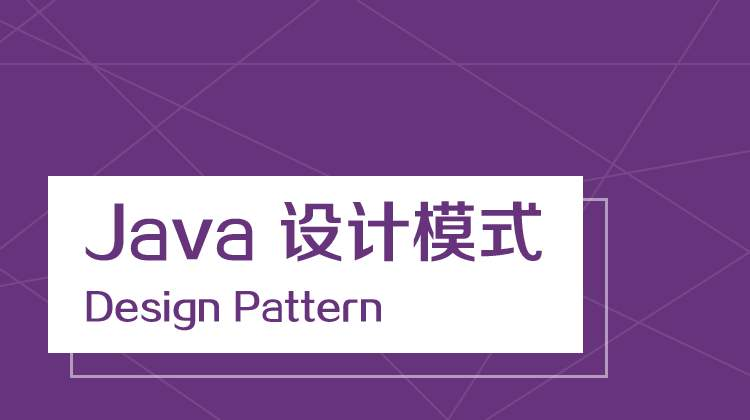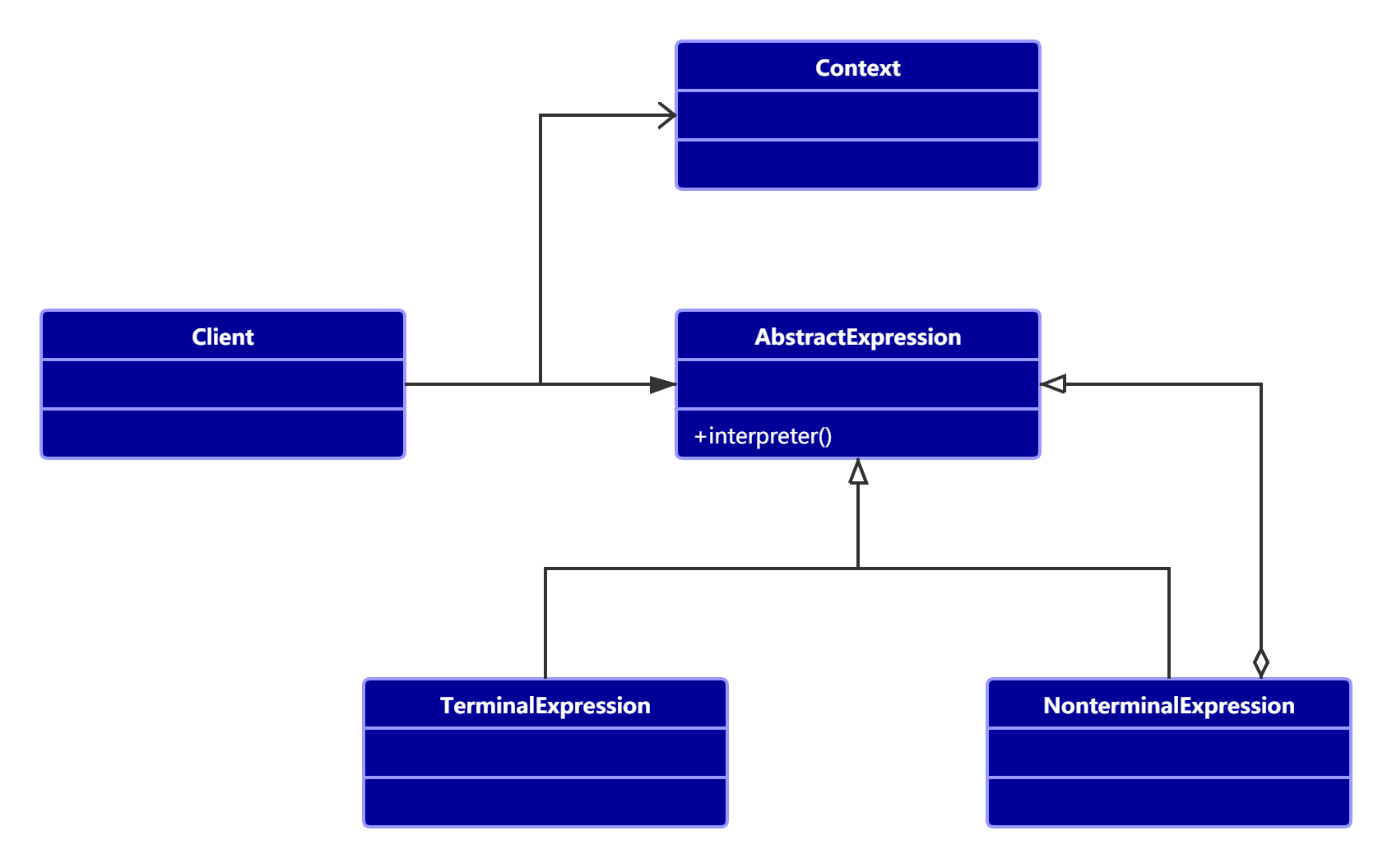# 「补课」进行时：设计模式(20)——解释器模式「补课」进行时：设计模式系列

## 2. 解释器模式

### 2.1 定义

Given a language, define a representation for its grammar along with an
interpreter that uses the representation to interpret sentences in the language.（给定一门语言， 定义它的文法的一种表示， 并定义一个解释器， 该解释器使用该表示来解释语言中的句子。 ）

### 2.2 通用类图• AbstractExpression 抽象解释器：具体的解释任务由各个实现类完成， 具体的解释器分别由 TerminalExpression 和 NonterminalExpression 完成。
• TerminalExpression 终结符表达式：实现与文法中的元素相关联的解释操作， 通常一个解释器模式中只有一个终结符表达式， 但有多个实例， 对应不同的终结符。
• NonterminalExpression 非终结符表达式：文法中的每条规则对应于一个非终结表达式， 具体到我们的例子就是加减法规则分别对应到 AddExpression 和 SubExpression 两个类。 非终结符表达式根据逻辑的复杂程度而增加， 原则上每个文法规则都对应一个非终结符表达式。
• Context 环境角色

### 2.3 通用代码

public abstract class Expression {
abstract Object interpreter(Context ctx);
}


public class TerminalExpression extends Expression {
// 通常终结符表达式只有一个， 但是有多个对象
public Object interpreter(Context context) {
return null;
}
}


public class NonterminalExpression extends Expression {
// 每个非终结符表达式都会对其他表达式产生依赖
public NonterminalExpression(Expression ...expressions) {
}
@Override
Object interpreter(Context ctx) {
// 进行文法处理
return null;
}
}


public class Client {
public static void main(String[] args) {
Context ctx = new Context();
Stack<Expression> stack = null;
for(int i = 0; i < args.length; i++){
// 进行语法判断， 并产生递归调用
}
// 产生一个完整的语法树， 由各个具体的语法分析进行解析
Expression exp = stack.pop();
//具体元素进入场景
exp.interpreter(ctx);
}
}


### 2.5 缺点

• 解释器模式会引起类膨胀。
• 解释器模式采用递归调用方法，将会导致调试非常复杂。
• 使用了大量的循环和递归，效率是一个不容忽视的问题。

## 3. 四则运算

public class Calculator {
private Expression expression;

// 构造函数，传参并解析
public Calculator(String expStr) {
// 安排运算先后顺序
Stack<Expression> stack = new Stack<>();
// 表达式拆分为字符数组
char[] charArray = expStr.toCharArray();

Expression left = null;
Expression right = null;

for(int i=0; i<charArray.length; i++) {
switch (charArray[i]) {
case '+':
left = stack.pop();
right = new VarExpression(String.valueOf(charArray[++i]));
stack.push(new AddExpression(left, right));
break;
case '-':
left = stack.pop();
right = new VarExpression(String.valueOf(charArray[++i]));
stack.push(new SubExpression(left, right));
break;
default :
stack.push(new VarExpression(String.valueOf(charArray[i])));
break;
}
}
this.expression = stack.pop();
}

public int run(HashMap<String, Integer> var) {
return this.expression.interpreter(var);
}
}


public abstract class Expression {
abstract int interpreter(HashMap<String, Integer> var);
}


public class VarExpression extends Expression {
private String key;
public VarExpression(String key) {
this.key = key;
}
@Override
int interpreter(HashMap<String, Integer> var) {
return var.get(this.key);
}
}


public class SymbolExpression extends Expression {
protected Expression left;
protected Expression right;

public SymbolExpression(Expression left, Expression right) {
this.left = left;
this.right = right;
}
@Override
int interpreter(HashMap<String, Integer> var) {
return 0;
}
}


public class AddExpression extends SymbolExpression {
public AddExpression(Expression left, Expression right) {
super(left, right);
}

@Override
int interpreter(HashMap<String, Integer> var) {
return super.left.interpreter(var) + super.right.interpreter(var);
}
}

public class SubExpression extends SymbolExpression {
public SubExpression(Expression left, Expression right) {
super(left, right);
}

@Override
int interpreter(HashMap<String, Integer> var) {
return super.left.interpreter(var) - super.right.interpreter(var);
}
}


public class Client {
public static void main(String[] args) throws IOException {
String expStr = getExpStr();
HashMap<String, Integer> var = getValue(expStr);
Calculator calculator = new Calculator(expStr);
System.out.println("运算结果：" + expStr + "=" + calculator.run(var));
}

public static String getExpStr() throws IOException {
System.out.print("请输入表达式：");
return (new BufferedReader(new InputStreamReader(System.in))).readLine();
}

public static HashMap<String, Integer> getValue(String expStr) throws IOException {
HashMap<String, Integer> map = new HashMap<>();
for(char ch : expStr.toCharArray()) {
if(ch != '+' && ch != '-' ) {
if(! map.containsKey(String.valueOf(ch))) {
System.out.print("请输入" + String.valueOf(ch) + "的值：");
String in = (new BufferedReader(new InputStreamReader(System.in))).readLine();
map.put(String.valueOf(ch), Integer.valueOf(in));
}
}
}
return map;
}
}


请输入表达式：a+b-c12-13159201-01149
04-303199
05-181万+
12-242128
04-121114
01-21504
09-2574
07-193482
09-15605
03-143783
03-161935
06-151万+
©️2020 CSDN 皮肤主题: 代码科技 设计师:Amelia_0503点击重新获取扫码支付1.余额是钱包充值的虚拟货币，按照1:1的比例进行支付金额的抵扣。
2.余额无法直接购买下载，可以购买VIP、C币套餐、付费专栏及课程。余额充值